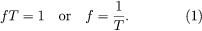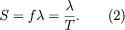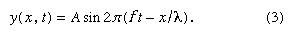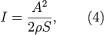# Sound

physics

Sound, a mechanical disturbance from a state of equilibrium that propagates through an elastic material medium. A purely subjective definition of sound is also possible, as that which is perceived by the ear, but such a definition is not particularly illuminating and is unduly restrictive, for it is useful to speak of sounds that cannot be heard by the human ear, such as those that are produced by dog whistles or by sonar equipment.lighthouse: Sound signals
The limitations of purely visual navigation very early led to the idea of supplementary audible warning in lighthouses. The first sound…

The study of sound should begin with the properties of sound waves. There are two basic types of wave, transverse and longitudinal, differentiated by the way in which the wave is propagated. In a transverse wave, such as the wave generated in a stretched rope when one end is wiggled back and forth, the motion that constitutes the wave is perpendicular, or transverse, to the direction (along the rope) in which the wave is moving. An important family of transverse waves is generated by electromagnetic sources such as light or radio, in which the electric and magnetic fields constituting the wave oscillate perpendicular to the direction of propagation.

Sound propagates through air or other mediums as a longitudinal wave, in which the mechanical vibration constituting the wave occurs along the direction of propagation of the wave. A longitudinal wave can be created in a coiled spring by squeezing several of the turns together to form a compression and then releasing them, allowing the compression to travel the length of the spring. Air can be viewed as being composed of layers analogous to such coils, with a sound wave propagating as layers of air “push” and “pull” at one another much like the compression moving down the spring.

A sound wave thus consists of alternating compressions and rarefactions, or regions of high pressure and low pressure, moving at a certain speed. Put another way, it consists of a periodic (that is, oscillating or vibrating) variation of pressure occurring around the equilibrium pressure prevailing at a particular time and place. Equilibrium pressure and the sinusoidal variations caused by passage of a pure sound wave (that is, a wave of a single frequency) are represented in Figure 1A and 1B, respectively.

## Plane waves

A discussion of sound waves and their propagation can begin with an examination of a plane wave of a single frequency passing through the air. A plane wave is a wave that propagates through space as a plane, rather than as a sphere of increasing radius. As such, it is not perfectly representative of sound (see below Circular and spherical waves). A wave of single frequency would be heard as a pure sound such as that generated by a tuning fork that has been lightly struck. As a theoretical model, it helps to elucidate many of the properties of a sound wave.

## Wavelength, period, and frequency

Figure 1C is another representation of the sound wave illustrated in Figure 1B. As represented by the sinusoidal curve, the pressure variation in a sound wave repeats itself in space over a specific distance. This distance is known as the wavelength of the sound, usually measured in metres and represented by λ. As the wave propagates through the air, one full wavelength takes a certain time period to pass a specific point in space; this period, represented by T, is usually measured in fractions of a second. In addition, during each one-second time interval, a certain number of wavelengths pass a point in space. Known as the frequency of the sound wave, the number of wavelengths passing per second is traditionally measured in hertz or kilohertz and is represented by f.

There is an inverse relation between a wave’s frequency and its period, such thatThis means that sound waves with high frequencies have short periods, while those with low frequencies have long periods. For example, a sound wave with a frequency of 20 hertz would have a period of 0.05 second (i.e., 20 wavelengths/second × 0.05 second/wavelength = 1), while a sound wave of 20 kilohertz would have a period of 0.00005 second (20,000 wavelengths/second × 0.00005 second/wavelength = 1). Between 20 hertz and 20 kilohertz lies the frequency range of hearing for humans. The physical property of frequency is perceived physiologically as pitch, so that the higher the frequency, the higher the perceived pitch. There is also a relation between the wavelength of a sound wave, its frequency or period, and the speed of the wave (S), such that## Mathematical values

The equilibrium value of pressure, represented by the evenly spaced lines in Figure 1A and by the axis of the graph in Figure 1C, is equal to the atmospheric pressure that would prevail in the absence of the sound wave. With passage of the compressions and rarefactions that constitute the sound wave, there would occur a fluctuation above and below atmospheric pressure. The magnitude of this fluctuation from equilibrium is known as the amplitude of the sound wave; measured in pascals, or newtons per square metre, it is represented by the letter A. The displacement or disturbance of a plane sound wave can be described mathematically by the general equation for wave motion, which is written in simplified form as:This equation describes a sinusoidal wave that repeats itself after a distance λ moving to the right (+ x) with a velocity given by equation (2).

The amplitude of a sound wave determines its intensity, which in turn is perceived by the ear as loudness. Acoustic intensity is defined as the average rate of energy transmission per unit area perpendicular to the direction of propagation of the wave. Its relation with amplitude can be written aswhere ρ is the equilibrium density of the air (measured in kilograms per cubic metre) and S is the speed of sound (in metres per second). Intensity (I) is measured in watts per square metre, the watt being the standard unit of power in electrical or mechanical usage.

The value of atmospheric pressure under “standard atmospheric conditions” is generally given as about 105 pascals, or 105 newtons per square metre. The minimum amplitude of pressure variation that can be sensed by the human ear is about 10-5 pascal, and the pressure amplitude at the threshold of pain is about 10 pascals, so the pressure variation in sound waves is very small compared with the pressure of the atmosphere. Under these conditions a sound wave propagates in a linear manner—that is, it continues to propagate through the air with very little loss, dispersion, or change of shape. However, when the amplitude of the wave reaches about 100 pascals (approximately one one-thousandth the pressure of the atmosphere), significant nonlinearities develop in the propagation of the wave.

Nonlinearity arises from the peculiar effects on air pressure caused by a sinusoidal displacement of air molecules. When the vibratory motion constituting a wave is small, the increase and decrease in pressure are also small and are very nearly equal. But when the motion of the wave is large, each compression generates an excess pressure of greater amplitude than the decrease in pressure caused by each rarefaction. This can be predicted by the ideal gas law, which states that increasing the volume of a gas by one-half decreases its pressure by only one-third, while decreasing its volume by one-half increases the pressure by a factor of two. The result is a net excess in pressure—a phenomenon that is significant only for waves with amplitudes above about 100 pascals.

Sound

## External Websites

Britannica Websites
Articles from Britannica Encyclopedias for elementary and high school students.

## Article Contributors

×
Britannica presents a time-travelling voice experience